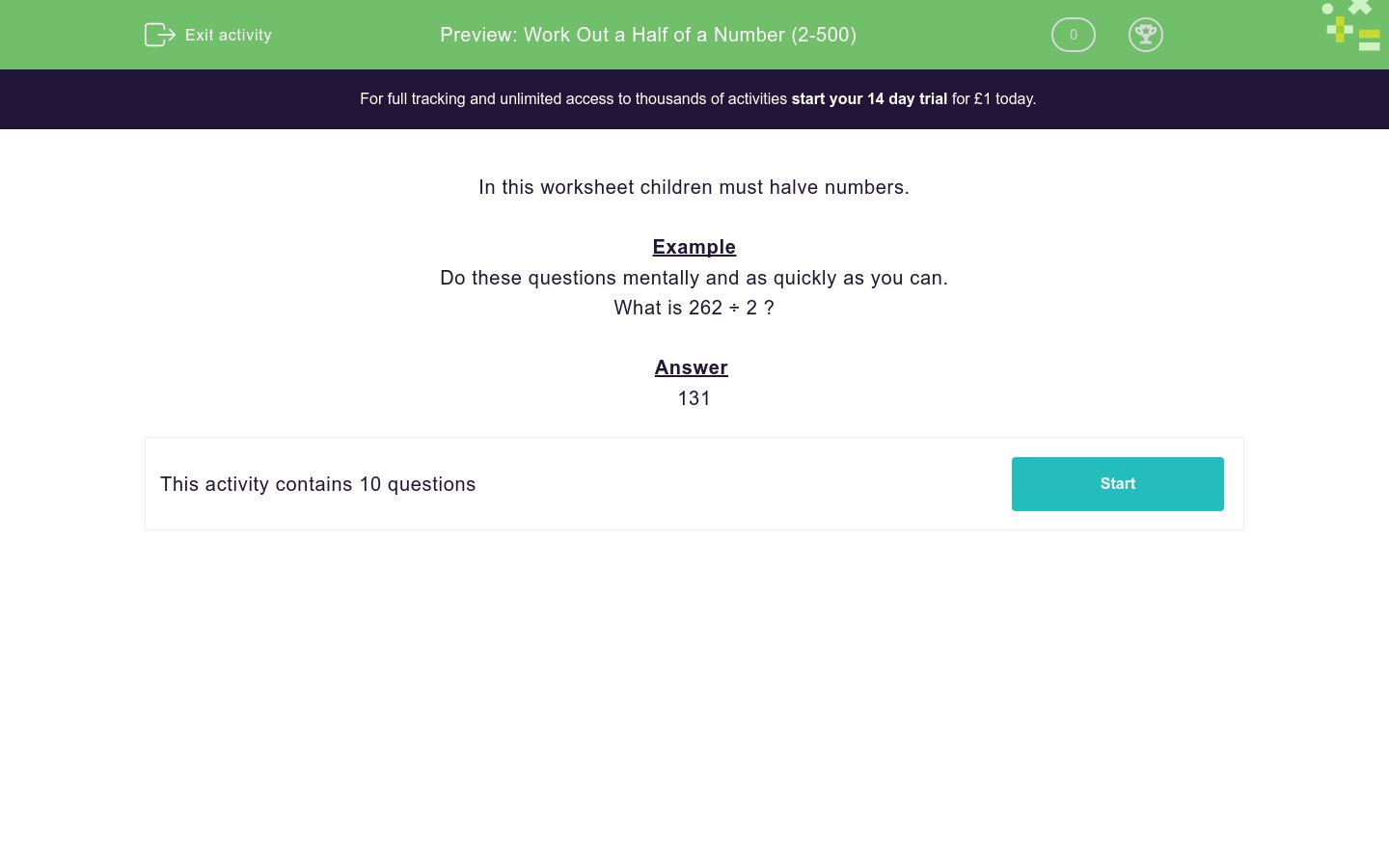# Work Out a Half of a Number (2-500)

In this worksheet, students use their division skills to halve numbers in their head by dividing by two.Key stage:  KS 2

Curriculum topic:   Number: Multiplication and Division

Curriculum subtopic:   Use Multiplication/Division Facts

Difficulty level:### QUESTION 1 of 10

In this worksheet children must halve numbers.

Example

Do these questions mentally and as quickly as you can.

What is 262 ÷ 2 ?

131

What is half of 44?

24

22

What is 86 ÷ 2 ?

44

43

What is half of 286?

143

134

What is 646 ÷ 2 ?

323

333

What is 288 ÷ 2 ?

144

188

What is 482 ÷ 2 ?

241

281

What is 140 ÷ 2 ?

70

10

What is 142 ÷ 2 ?

72

71

What is 166 ÷ 2 ?

88

83

What is 850 ÷ 2 ?

450

425

• Question 1

What is half of 44?

22
• Question 2

What is 86 ÷ 2 ?

43
• Question 3

What is half of 286?

143
• Question 4

What is 646 ÷ 2 ?

323
• Question 5

What is 288 ÷ 2 ?

144
• Question 6

What is 482 ÷ 2 ?

241
• Question 7

What is 140 ÷ 2 ?

70
• Question 8

What is 142 ÷ 2 ?

71
• Question 9

What is 166 ÷ 2 ?

83
• Question 10

What is 850 ÷ 2 ?